# DAV Class 3 Maths Chapter 12 Worksheet 4 Solutions

The DAV Maths Book Class 3 Solutions and DAV Class 3 Maths Chapter 12 Worksheet 4 Solutions of Geometry offer comprehensive answers to textbook questions.

## DAV Class 3 Maths Ch 12 WS 4 Solutions

Question 1.
Write the length of each of the following line segments by reading the scale.

(a)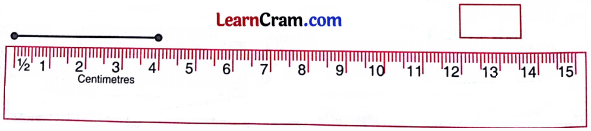Solution:
4 cm

(b)Solution:
6 cm

(c)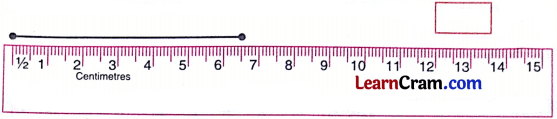Solution:
6.5 cm

(d)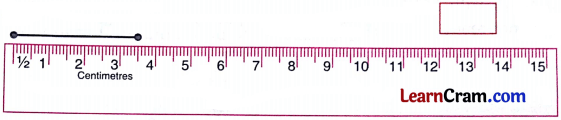Solution:
3.5 cmQuestion 2.
Joint the two points given in each question and measure the line segments with the help of a scale.

(a)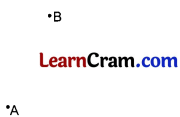Solution: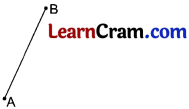(b)Solution: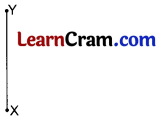(c)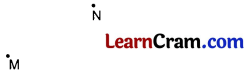Solution: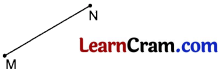Question 3.
Compare the given sets of line segments by measuring them using a scale.

(a)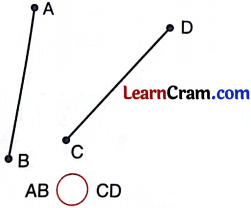Solution:
AB = 4.3 cm
CD = 4.5 cm
AB < CD

(b)Solution:
PQ = 4.3 cm
XY = 3.8 cm
XY < PQ

(c)Solution:
PQ = 3.8 cm
RS = 3.8 cm
PQ = RS### DAV Class 3 Maths Chapter 12 Worksheet 4 Notes

Measuring Line Segment

We make use of a 15 cm scale to measure and draw line segments.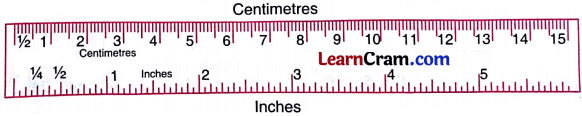Place the edge of centimetre scale along the given line AB. Remember to keep zero mark at A.
Here AB is 5.7 cm.